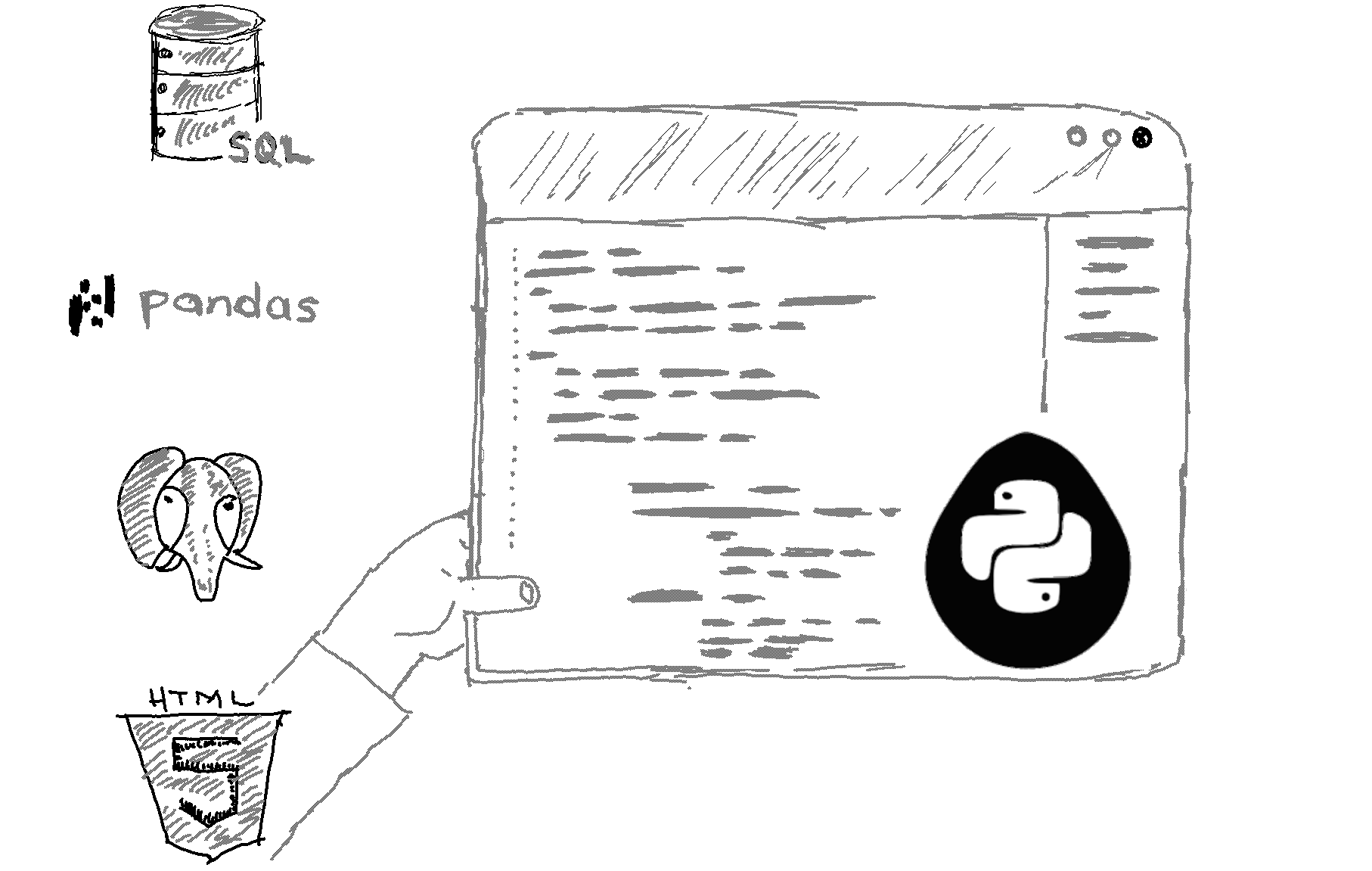# What is the difference between a staticmethod and a classmethod in Python?

First, let us look at a "normal" instance method:

```class Square:    sides = 4
def perimeter(self, x):        return x * self.sides
mysquare = Square()print(mysquare.perimeter(10))```

The perimeter method is an instance method here because it operates on an instance of the class Square (i.e., mysquare), which in our example has a side length of 10.

Now, let us look at a class method:

```class Square:    sides = 4
@classmethod    def angles(cls):        return cls.sides
print(Square. angles())```

Think of a class method as a method that processes information about the class (i.e., the number of sides of square types/classes). On the other hand, an instance method processes information specific to an instance of that class (i.e., the perimeter value of a particular square).

In other words, since all squares have four angles, if you want to process information about the number of angles, it would make more sense to do that in a class method. So, in Python, we create a class method by attaching the @classmethod Python decorator above the method definition.

Notice that we provided a cls parameter to our class method. That cls variable will contain the Square class. Therefore cls.sides is equivalent to Square.sides. So, what we did is we accessed the sides class variable from within our angles class method. Note that in instance methods, we use self as a parameter, and self will contain the square instance of the Square class (i.e., mysquare), not the Square class itself.

Take a look at the square_definition static method below:

```class Square:    sides = 4
@staticmethod    def square_definition():        return "A square is a regular quadrilateral shape."    print(Square.square_definition())```

A static method does not operate on the instance and does not operate on the class either. Instead, it is just a function that, for organizational reasons, you might decide to keep inside your class.

Difference between a class method and a static method

Well, class methods usually need to access an attribute of the class. For example, our class method accessed the sides class variable. Static methods don't care about the class attributes. They are just functions that we decide to keep inside a class since they are logically related to that class.

##### Recommended Course

Learn Flask development and learn to build cool apps with our premium Python course on Udemy.